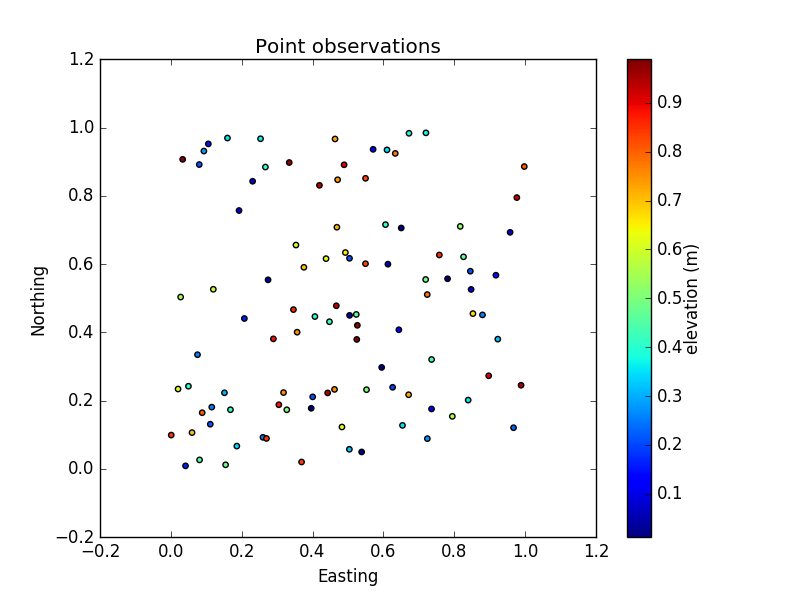# Scatter plots using matplotlib.pyplot.scatter()

First, let’s install pyplot from matplotlib and call it `plt`:

``````import matplotlib.pyplot as plt
``````

We are also going to need some data which we’ll create using numpy - type the following:

``````import numpy as np
``````

Now lets create some random point data to mimic some xy coordinates and some associated attribute:

``````xyz=np.array(np.random.random((100,3)))
``````

## The basic scatter

To create our plot, we are going to use the `plt.scatter()` function (remember to check out the function help by using `plt.scatter?`) - an alternative to `plt.plot()` which gives you more control on setting colours based on another variable. This function takes in 2 variables to plot - we’ll use the first 2 columns of our `xyz` array:

``````plt.scatter(xyz[:,0], xyz[:,1])
``````

You should see something like the following being printed out:

``````>>> <matplotlib.collections.PathCollection at 0x1afd1d30>
``````

To view the image, we can use the function `plt.show()` - type this in:

``````plt.show()
``````

and you should see something like this:## Improving appearance

This is all well and good but we are missing some important components - for example axis labels and a title. These are easily added - first you must re-create the scatter plot:

``````plt.scatter(xyz[:,0], xyz[:,1])
``````

Using the created `plt` instance, you can add labels like this:

``````plt.title("Point observations")
plt.xlabel("Easting")
plt.ylabel("Northing")
``````

If you’ve had a look at the documentation for `plt.scatter()` you will also see that the function can take in a scalar to adjust the marker size (starts at a default value of 20). To make these smaller, you must pass in a value to the `plt.scatter()` function:

``````marker_size=15
plt.scatter(xyz[:,0], xyz[:,1], marker_size)
``````

What we can also do is change the colour of the points - want them in red? Then when invoking `plt.scatter()`, you’ll need to set the `c` flag (more info on colours can be found here):

``````plt.scatter(xyz[:,0], xyz[:,1], c='r')
``````

Something else that can be handy is to colour the points by another variable - in the case of our `xyz` test data, currently we are only using the first 2 columns - lets use the third colmn to colour the plot:

``````plt.scatter(xyz[:,0], xyz[:,1], c=xyz[:,2])
``````

By adding these new colours, we now have information on the plot that alone is not particularly informative - we need a colorbar and fortunately, there is a method for creating this - `plt.colorbar()`:

``````plt.scatter(xyz[:,0], xyz[:,1], c=xyz[:,2])
plt.colorbar()

``````

Last but not least, lets add a title to the colorbar to indicate what it represents - to do this, after creating your initial plot, assign the creation of your colorbar to a variable like this:

``````cbar = plt.colorbar()
``````

You can now access methods of `colorbar` - have a look at what’s available by typing `cbar.` followed by the tab key.

To set the title of the colorbar, we need to type:

``````cbar.set_label("elevation (m)")
``````

You can change the distance the label is from the colorbar by using the labelpad option (positive moves away, negative moves it closer):

``````cbar.set_label("elevation (m)", labelpad=-1)
``````

Now, to create your final plot just type:

``````marker_size=15
plt.scatter(xyz[:,0], xyz[:,1], marker_size, c=xyz[:,2])
plt.title("Point observations")
plt.xlabel("Easting")
plt.ylabel("Northing")
cbar= plt.colorbar()Note - if you have created multiple scatter plots (i.e. have enterered `plt.scatter()` a few times with no call to `plt.show()`), then these will all be plotted visually on your call to `plt.show()`. If you are concerned that this is going to happen (and you only want to display your most recent `plt.scatter()` call), then type `plt.clf()` which clears everything, and then retype the code to create your figure.
Again, to be safe and ensure everything is clean, type `plt.clf()` again.